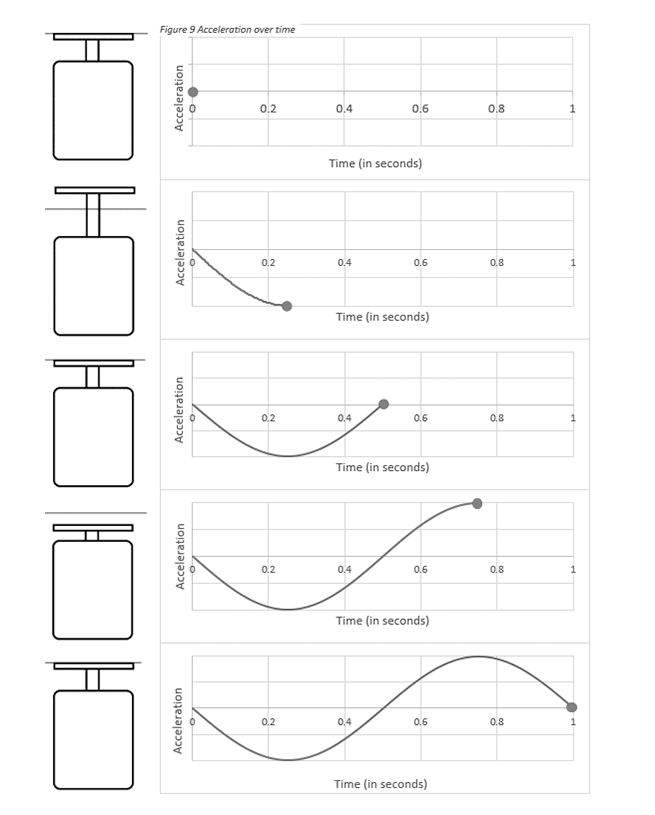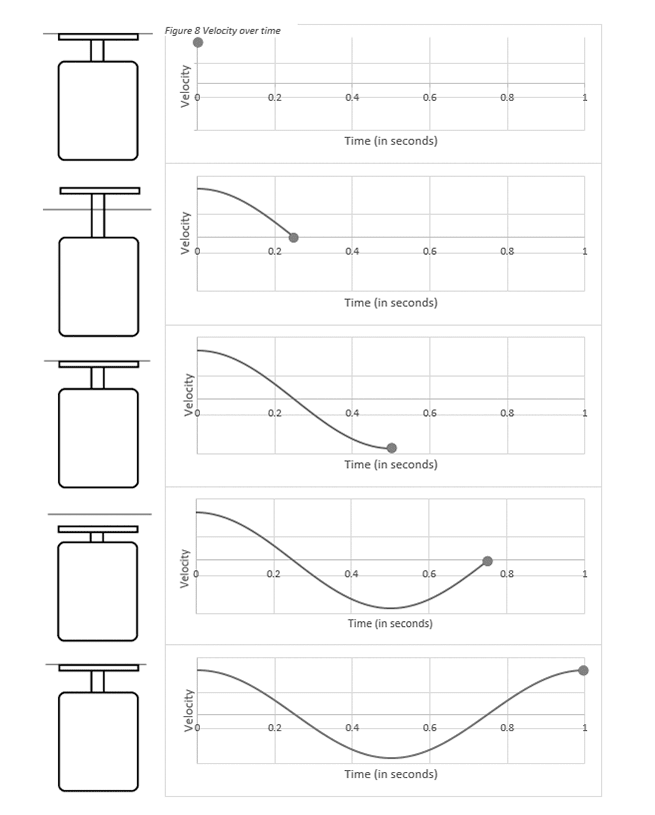# Acceleration, Velocity, and Displacement

March 29, 2018

During a sine vibration test, the device under test (DUT) is subject to excitation, and the system collects its vibrational response. This response waveform provides information about the DUT’s motion following an external excitation and helps identify the damage potential of the input vibration.

Acceleration, velocity, and displacement use the response waveform to measure the change in the object’s motion.

### Acceleration

Acceleration is the rate of change in velocity. When a car accelerates, its velocity increases. When it decelerates, its velocity decreases. What would a graph of acceleration over time look like? Consider the following: a wave has zero velocity at the crest of a cycle. Then, the wave moves downward at a negative velocity. It increases in negative velocity until it reaches the rest position; at which point, the wave begins to slow down.

During this time, the acceleration is negative because the velocity is increasing in a negative direction. However, once the wave is past the rest position, it slows down until it reaches a momentary standstill at the trough of the cycle. At this point, the velocity becomes positive and the wave moves upward.

In other words, when a wave passes the rest position, the velocity increases in the positive direction from negative to zero to positive velocity. Figure 2.1 depicts the acceleration of the wave over time.Figure 2.1. Acceleration over time.

Acceleration is the rate at which velocity changes and is measured in meters per second per second. We can think of it as the meters per second change in velocity every second. In vibration testing, acceleration uses the gravitational constant unit of G.

### Velocity

Velocity refers to the rate of change in the position of the DUT. At the highest point, or peak, of the cycle, the DUT is momentarily at a standstill and the velocity is zero. Then, it descends and picks up speed.

The DUT reaches the point of greatest negative velocity when it crosses the rest position; after which point, it begins to slow down. At the lowest point (trough) of the cycle, the DUT is again momentarily at a standstill and the velocity is zero. It begins the process again by climbing up and gaining positive speed.

Figure 2.2 displays velocity over time. The velocity is positive at the beginning as if the test was already in motion when the data was collected. Velocity is the rate at which position changes and is measured in meters per second.Figure 2.2. Velocity over time.

### Displacement

Displacement is the distance an object has moved expressed as units of length such as meters (m) or inches (in).

### Frequency Range in Sine Testing

Thus far, we have discussed single-tone sine tests at low frequencies. In reality, sine vibration testing takes place over a broad range of frequencies from 10 to 10,000 hertz (Hz). When we shake a DUT with a 5,000Hz sine tone, it undergoes 5,000 cycles every second.

Below is a slow-motion video showing the displacement and velocity of a shaker head vibrating at 5Hz. When the displacement is at the maximum or minimum point, the velocity of the shaker head is zero.